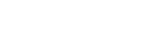## QUESTIONS

INSTRUCTIONS: Answer all questions in the spaces provided

1. Simplify(3mks)
2. Using mathematical tables, find the value of (3mks)
1. 456 × 397
273
2. 0.14683     1      (2mks)
27.38
3.
1. Determine the gradient and the y-intercept of the following equation (3mks)
1/3 x + 2/5 y+1/= 0
2. Find an equation perpendicular to the line 2y=3x+4 and passing through point (2, 6) (4mks)
4. The volume scale factor of two similar cylinders is 27. Find;
1. The linear scale factor (2mks)
2. The area scale factor (1mk)
5. By substitution method, solve the following simultaneous equations
2x + 4y = 16
3x - y = 2 (3mks)
6. A quadrilateral has vertices P(-3,7), Q(-5,3), R(-3,1), and S(-1,3).
1. On the grid provided, draw the quadrilateral and name it (2mks)2. Find the equation of the line of symmetry of the quadrilateral formed in (a) above. (2mks)
3. Draw the image of quadrilateral PQRS under a reflection in the line y=x and give the coordinates of the image. (3mks)
4. Find the equation of the line of symmetry of the image (2mks)

## MARKING SCHEME

INSTRUCTIONS: Answer all questions in the spaces provided

1. Simplify(3mks)
(a × a × a × a)  × ( b × b × b× (a × a) × (c × c × c  × c × (b-1  × b-1) × (c × c × c)
(a-1 × a-1 × a-1) × (b × × b × (c ×× c)
a3× a2× c4× b-2
a-3
2. Using mathematical tables, find the value of (3mks)
1. 456 × 397
273
 No Std form Log 456 4.56 × 102 2.6590 397 3.97 × 102 + 2.5988 - 5.2578 273 2.73 × 102 2.4362 2.8216
2.8216
0.8216 × 102
Anilog of 0.8216
= 6.63132 × 102
= 663.132
2. 0.14683     1      (2mks)
27.38
 No Std Form Log 0.14683 (1.468 × 10-1)3 3.1635 × 10-3 1/27.38 1/2.738 × 101 1/10 × 0.3655
= 0.03655
+ 0.003165
0.039715
= 0.039715
3.
1. Determine the gradient and the y-intercept of the following equation (3mks)
1/3 x + 2/5 y+1/= 0
5/2 × 2/5y = -1/6 × 5/2 - 1/3x × 5/2
y = (-1/6 × 5/8) - 1/3x × 5/2
y = 5/48 - 5/6x
2. Find an equation perpendicular to the line 2y=3x+4 and passing through point (2, 6) (4mks)
M1M2 = -1
2y = 3x + 4
2      2      2
y = 3/2x + 2
M1 = 3/2
2/3 × 3/2 × m2 = -1 × 2/3
m2 = -2/3
y - 6 = 2
x - 2    3
3y - 18 = -2x + 4
3y = 2x + 22
3      3      3
y = -2/3x + 22/3
4. The volume scale factor of two similar cylinders is 27. Find;
1. The linear scale factor (2mks)
3√27 = 3
L.S.F = 3
2. The area scale factor (1mk)
A.S.F = (L.S.F)2
32 = 9
5. By substitution method, solve the following simultaneous equations
2x + 4y = 16
3x - y = 2 (3mks)
2x(2x + 4(3x - 2)) = 16
2x + 12x - 18 = 16
14x = 24
14     14
x = 12/7
y = 3x - 2
y = (3 × 12/7) - 2
y = 36/7 - 2/1
y = 36 - 14
7
y = 22/7
6. A quadrilateral has vertices P(-3,7), Q(-5,3), R(-3,1), and S(-1,3).
1. On the grid provided, draw the quadrilateral and name it (2mks)
kite2. Find the equation of the line of symmetry of the quadrilateral formed in (a) above. (2mks)
P(-3,7) and R(-3,1)
3. Draw the image of quadrilateral PQRS under a reflection in the line y=x and give the coordinates of the image. (3mks)
P1 = (7, -3)
Q1 = (3, -5)
R1 = (1, -3)
S1 = (3, -1)
4. Find the equation of the line of symmetry of the image (2mks)
P(7, -3)
R(1,-3)

• ✔ To read offline at any time.
• ✔ To Print at your convenience
• ✔ Share Easily with Friends / Students